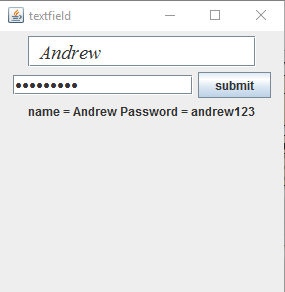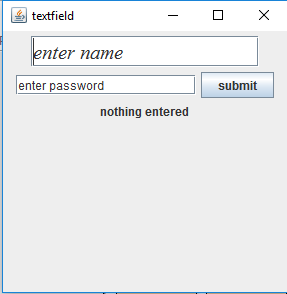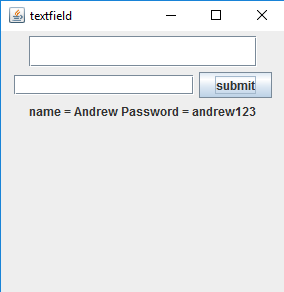Related Articles
Java Swing | JPasswordField
• Last Updated : 14 Apr, 2021

PasswordField is a part of javax.swing package . The class JPasswordField is a component that allows editing of a single line of text where the view indicates that something was typed by does not show the actual characters. JPasswordField inherits the JTextField class in javax.swing package.
Constructors of the class are :

1. JPasswordField(): constructor that creates a new PasswordField

2. JPasswordField(int columns) : constructor that creates a new empty PasswordField with specified number of columns.

3. JPasswordField(String Password) : constructor that creates a new empty Password field initialized with the given string.

4. JPasswordField(String Password, int columns) : constructor that creates a new empty PasswordField with the given string and a specified number of columns .

5. JPasswordField(Document doc, String Password, int columns) : constructor that creates a Passwordfield that uses the given text storage model and the given number of columns.

Commonly used method of JPasswordField :

1. char getEchoChar() : returns the character used for echoing in JPasswordField.

2. setEchoChar(char c) : set the echo character for JPasswordField.

3. String getPassword() : returns the text contained in JPasswordField.

4. String getText() : returns the text contained in JPasswordField.

1. Program to enter name and password using JTextField and JPasswordField

## Java

 `// Java Program to enter name and password``// using JTextField and JPasswordField``import` `java.awt.event.*;``import` `java.awt.*;``import` `javax.swing.*;``class` `text ``extends` `JFrame ``implements` `ActionListener {``    ``// JTextField``    ``static` `JTextField t;` `    ``// JPasswordField``    ``static` `JPasswordField pass;` `    ``// JFrame``    ``static` `JFrame f;` `    ``// JButton``    ``static` `JButton b;` `    ``// label to display text``    ``static` `JLabel l;` `    ``// default constructor``    ``text()``    ``{``    ``}` `    ``// main class``    ``public` `static` `void` `main(String[] args)``    ``{``        ``// create a new frame to store text field and button``        ``f = ``new` `JFrame(``"textfield"``);` `        ``// create a label to display text``        ``l = ``new` `JLabel(``"nothing entered"``);` `        ``// create a new button``        ``b = ``new` `JButton(``"submit"``);` `        ``// create a object of the text class``        ``text te = ``new` `text();` `        ``// addActionListener to button``        ``b.addActionListener(te);` `        ``// create a object of JTextField with 16 columns and initial text``        ``t = ``new` `JTextField(``"enter name"``, ``16``);` `        ``// create a object of passwordField with 16 columns``        ``pass = ``new` `JPasswordField(``16``);` `        ``// create an object of font type``        ``Font fo = ``new` `Font(``"Serif"``, Font.ITALIC, ``20``);` `        ``// set the font of the textfield``        ``t.setFont(fo);` `        ``// create a panel to add buttons and textfield``        ``JPanel p = ``new` `JPanel();` `        ``// add buttons and textfield to panel``        ``p.add(t);``        ``p.add(pass);``        ``p.add(b);``        ``p.add(l);` `        ``// add panel to frame``        ``f.add(p);` `        ``// set the size of frame``        ``f.setSize(``300``, ``300``);` `        ``f.show();``    ``}` `    ``// if the button is pressed``    ``public` `void` `actionPerformed(ActionEvent e)``    ``{``        ``String s = e.getActionCommand();``        ``if` `(s.equals(``"submit"``)) {``            ``// set the text of the label to the text of the field``            ``l.setText(``"name = "` `+ t.getText() + ``"\t Password = "` `+ pass.getText());` `            ``// set the text of field to blank``            ``t.setText(``"  "``);` `            ``// set the text of password field to blank``            ``pass.setText(``""``);``        ``}``    ``}``}`

output :2. Program to change the echo character of JPasswordField and set initial text for password field

## Java

 `// Java Program to change the echo character of``// JPasswordField and set initial text for password field``import` `java.awt.event.*;``import` `java.awt.*;``import` `javax.swing.*;``class` `text ``extends` `JFrame ``implements` `ActionListener, FocusListener {``    ``// JTextField``    ``static` `JTextField t;` `    ``// JPasswordField``    ``static` `JPasswordField pass;` `    ``// JFrame``    ``static` `JFrame f;` `    ``// JButton``    ``static` `JButton b;` `    ``// label to display text``    ``static` `JLabel l;` `    ``// default constructor``    ``text()``    ``{``    ``}` `    ``// main class``    ``public` `static` `void` `main(String[] args)``    ``{``        ``// create a new frame to store text field and button``        ``f = ``new` `JFrame(``"textfield"``);` `        ``// create a label to display text``        ``l = ``new` `JLabel(``"nothing entered"``);` `        ``// create a new button``        ``b = ``new` `JButton(``"submit"``);` `        ``// create a object of the text class``        ``text te = ``new` `text();` `        ``// addActionListener to button``        ``b.addActionListener(te);` `        ``// create a object of JTextField with 16 columns and initial text``        ``t = ``new` `JTextField(``"enter name"``, ``16``);` `        ``// create a object of passwordField with 16 columns``        ``pass = ``new` `JPasswordField(``16``);` `        ``// add FocusListener to passwordField``        ``pass.addFocusListener(te);` `        ``// set the echo character of the password field``        ``pass.setEchoChar((``char``)``0``);` `        ``// set initial text for password field``        ``pass.setText(``"enter password"``);` `        ``// set the echo character of the password field``        ``// create an object of font type``        ``Font fo = ``new` `Font(``"Serif"``, Font.ITALIC, ``20``);` `        ``// set the font of the textfield``        ``t.setFont(fo);` `        ``// create a panel to add buttons and textfield``        ``JPanel p = ``new` `JPanel();` `        ``// add buttons and textfield to panel``        ``p.add(t);``        ``p.add(pass);``        ``p.add(b);``        ``p.add(l);` `        ``// add panel to frame``        ``f.add(p);` `        ``// set the size of frame``        ``f.setSize(``300``, ``300``);` `        ``f.show();``    ``}``    ``// flag to set the text to blank for the first time when the component gets focus``    ``boolean` `flag = ``true``;` `    ``// events of focus listener``    ``// when focus is gained``    ``public` `void` `focusGained(FocusEvent e)``    ``{``        ``if` `(flag) {``            ``// set a definite echo char``            ``pass.setEchoChar(``'*'``);` `            ``// only set the text to blank for 1st time``            ``// set the text to blank``            ``pass.setText(``""``);``            ``flag = ``false``;``        ``}``    ``}` `    ``// when the focus is lost``    ``public` `void` `focusLost(FocusEvent e)``    ``{``    ``}` `    ``// if the button is pressed``    ``public` `void` `actionPerformed(ActionEvent e)``    ``{``        ``String s = e.getActionCommand();``        ``if` `(s.equals(``"submit"``)) {``            ``// set the text of the label to the text of the field``            ``l.setText(``"name = "` `+ t.getText() + ``"\t, Password = "` `+ pass.getText());` `            ``// set the text of field to blank``            ``t.setText(``"  "``);` `            ``// set the text of password field to blank``            ``pass.setText(``""``);``        ``}``    ``}``}`Note : The above programs might not run in an online compiler use an offline IDE.
the default text and number of columns of password can be changed by the programmer as per their need.

Attention reader! Don’t stop learning now. Get hold of all the important Java Foundation and Collections concepts with the Fundamentals of Java and Java Collections Course at a student-friendly price and become industry ready. To complete your preparation from learning a language to DS Algo and many more,  please refer Complete Interview Preparation Course.

My Personal Notes arrow_drop_up